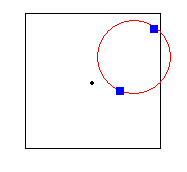### Problem 1: Lion in a Circular Cage Puzzle

Source: Asked to me by Pramod Ganapathi (PhD Student at Stony Brook University)

Problem:
A lion and a lion tamer are enclosed within a circular cage. If they move at the same speed but are both restricted by the cage, can the lion catch the lion tamer? (Represent the cage by a circle, and the lion and lion tamer as two point masses within it.)

### Problem 2: Geometry Puzzle: Center of Square in CircleSource: Asked to me by a lot of people from IIT Bombay on email and posted by a few on "Post a Question" page.

Problem:
What is the probability that two uniform random points (to be precise: i.i.d. with respect to Lebesgue measure) in the square are such that center of the square lies in the circle formed by taking the points as diameter.

Let's say A keep tossing a fair coin, until he get 2 consecutive heads, define X to be the number of tosses for this process; B keep tossing another fair coin, until he get 3 consecutive heads, define Y to be the number of the tosses for this process.

1) Calculate P{X>Y}

2) What's the expected value of X

3) What's the expected value of Y

### Problem 4: Coins Puzzle

There are 100 coins on the table out of which 50 are tail-face up and 50 are head face up. You are blind folded and there is no way to determine which side is up by rubbing, etc. You have to divide the 100 coins in two equal halves such that both have equal number of coins with tails face up. (This obviously implies that the two have equal number of coins with heads face up)

Second part: There are 100 coins on the table out of which 10 are tail-face up and 90 are head face up. You are blind folded and there is no way to determine which side is up by rubbing, etc. You have to divide the 100 coins in two halves (not necessarily equal) such that both have equal number of coins with tails face up.

### Problem 5: Arithmetic Puzzle: Broken Calculator

Source: Quantnet ForumProblem:
There is a calculator in which all digits(0-9) and the basic arithmetic operators(+,-,*,/) are disabled. However other scientific functions are operational like exp, log, sin, cos, arctan, etc. The calculator currently displays a 0. Convert this first to 2 and then to 3.